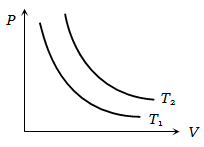NEET Physics Kinetic Theory of Gases Questions Solved

The adjoining figure shows graph of pressure and volume of a gas at two temperatures ${\mathrm{T}}_{1}$ and ${\mathrm{T}}_{2}$. Which of the following interferences is correct1.  ${\mathrm{T}}_{1}>{\mathrm{T}}_{2}$

2. ${\mathrm{T}}_{1}={\mathrm{T}}_{2}$

3. ${\mathrm{T}}_{1}<{\mathrm{T}}_{2}$

4. No interference can be drawn

Explanation is a part of a Paid Course. To view Explanation Please buy the course.

Difficulty Level: